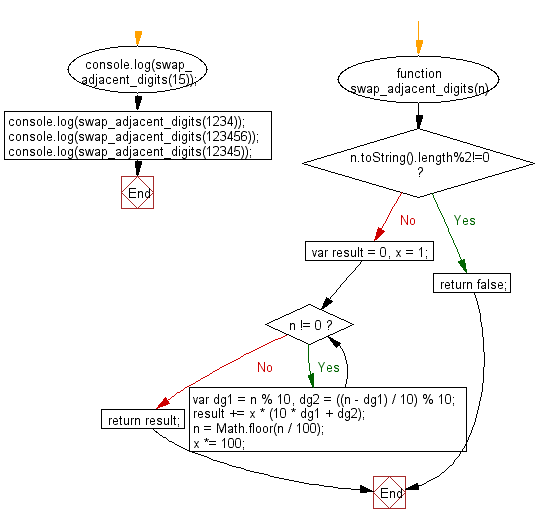﻿ C Program: Change the array elements - w3resource

# C Exercises: Change the array elements

## C Basic Declarations and Expressions: Exercise-127 with Solution

Write a C program that reads an array of integers (length 8), replaces the 1st element with the 8th, the 2nd with the 7th and so on. Print the final array.

Sample Solution:

C Code:

``````#include <stdio.h>
int main () {
unsigned short i, j; // Declare variables for looping
short array_nums, n, temp1, temp2; // Declare array and temporary variables

// Prompt user for input
printf("Input 8 array elements:\n");

// Loop to read 8 short values from the user and store them in the array
for (i = 0; i < 8; i++) {
scanf("%hd", &n);
array_nums[i] = n;
}

// Loop to swap elements from the first half of the array with the second half
for (i = 0, j = 7; i <= 4 && j >= 4; i++, j--) {
temp1 = array_nums[i];
temp2 = array_nums[j];
array_nums[i] = temp2;
array_nums[j] = temp1;
}

// Print modified array
printf("\nModified array:\n");
for (i = 0; i < 8; i++)
printf("array_nums[%d] = %d\n", i, array_nums[i]);

return 0; // End of program
}
``````

Sample Output:

```Input 8 array elements:
25
35
17
-5
29
45
60
65

Modified array:
array_nums = 65
array_nums = 60
array_nums = 45
array_nums = 29
array_nums = -5
array_nums = 17
array_nums = 35
array_nums = 25
```

Flowchart:C programming Code Editor:

What is the difficulty level of this exercise?

Test your Programming skills with w3resource's quiz.

﻿